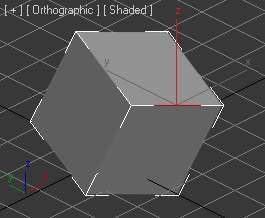This is now works (not right)This how to needСODE:

fn WorldToScreenPos_fn pos =
(
width=gw.getWinSizeX()
height=gw.getWinSizeY()

thePos = pos * viewport.getTM()
screenOrigin = mapScreenToView [0,0] (thePos.z) [width,height]

worldSize = screenOrigin*2
xAspect = width/(abs worldSize.x)
yAspect = height/(abs worldSize.y)

screenPos=point2 (xAspect *(thePos.x-screenOrigin.x)) (-(yAspect*(thePos.y-screenOrigin.y)))
if (screenPos.x) < 0 then (screenPos.x=0)
if (screenPos.y) < 0 then (screenPos.y=0)
screenPos.x = screenPos.x as integer
screenPos.y = screenPos.y as integer
[screenPos.x,screenPos.y]
)
fn ViewRotationObj_fn=
(
ang=0
vert = (PolyOp.GetVertsUsingEdge \$ (\$.getSelection #Edge)) as array
p1 = WorldToScreenPos_fn (\$.GetVertex vert)
p2 = WorldToScreenPos_fn (\$.GetVertex vert)
vectDirect = normalize (if p1.y > p2.y then (p1 - p2) else (p2 - p1))
horizont = (inverse(getViewTM())).row1
ang = acos (dot [vectDirect.x,vectDirect.y,0] horizont)
vectViewport = (inverse(getViewTM())).row3
rotate \$ (angleaxis ang vectViewport)
)
select (converttopoly (box())); subobjectlevel=2
\$.EditablePoly.SetSelection #Edge #{5}
ViewRotationObj_fn()